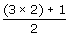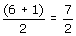## Definition Of Improper Fraction

An improper fraction is a fraction in which the number in the numerator is greater than or equal to the number in the denominator.

### Example of Improper Fraction

The following are few examples of improper fraction.
17/15, 9/1, 3/3

### Solved Example on Improper Fraction

#### Ques: Chris sharedof his homework with Valerie. Write this as an improper fraction.

##### Choices:

A. 1/6
B. 3/2
C. 7/2
D.2/7
Step 1:[Original mixed number.]
Step 3:[Multiply the whole number by denominator and add it to the numerator.]
Step 4:[Simplify.]